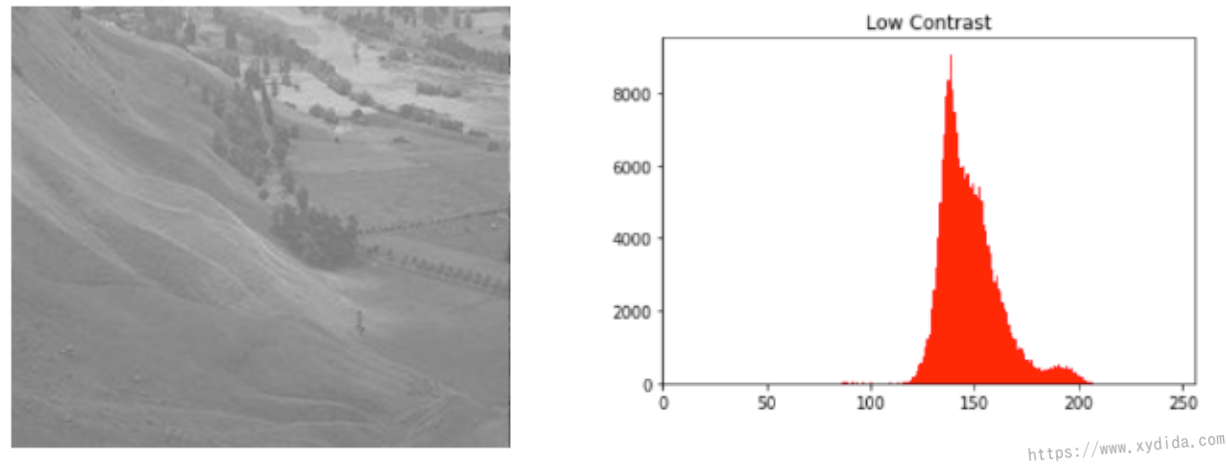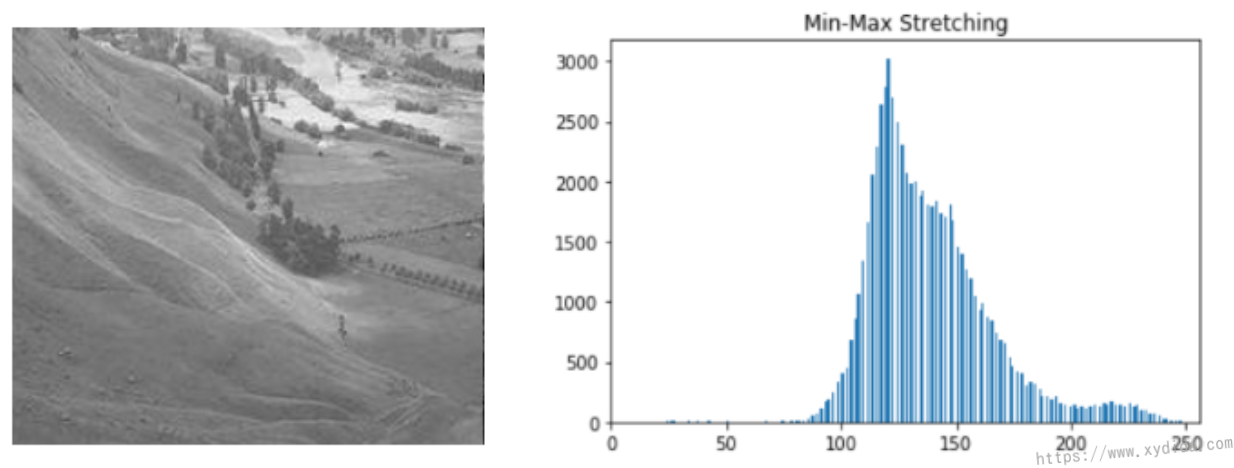# 关于对比度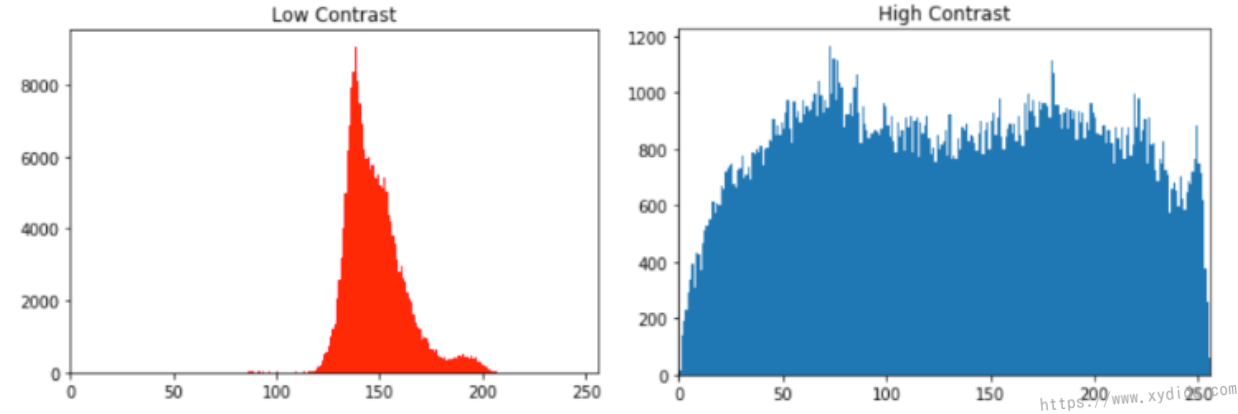# 如何提高对比度？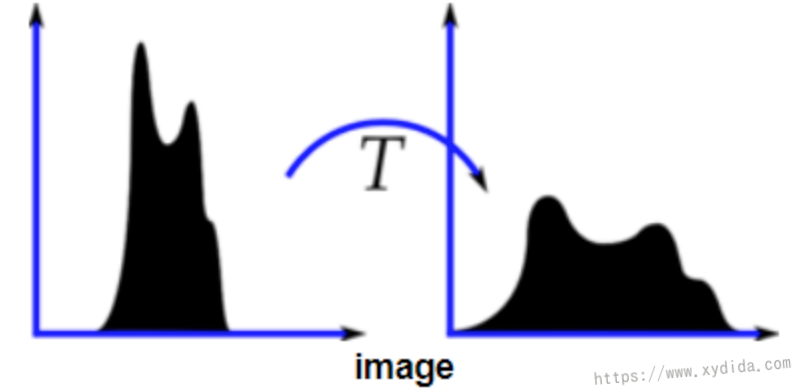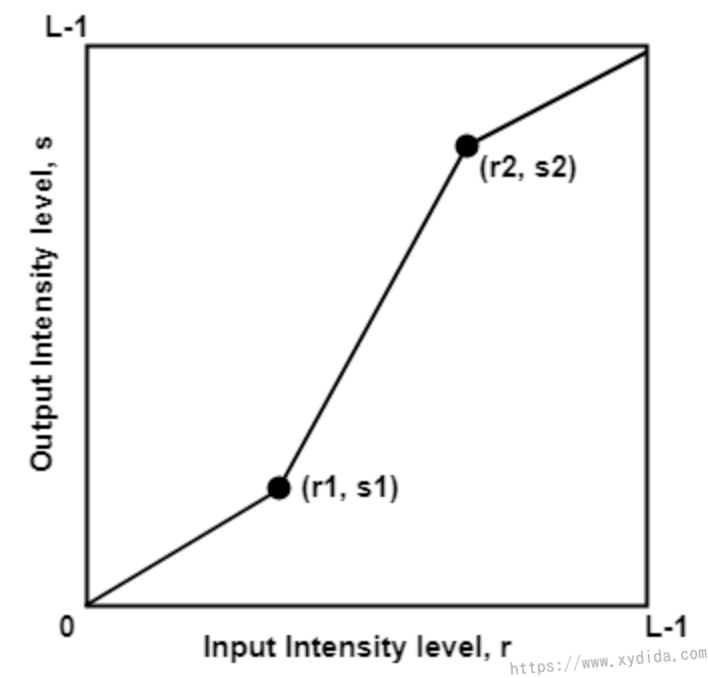⁃ 如果r1=r2, s1=s2, 则函数变成一个线性函数
⁃ 如果r1=r2, s1=0, s2=L-1, 则函数变成一个thresholding函数
⁃ 如果(r1,s1)=(r_min, 0), (r2, s2)=(r_max, L-1), 则函数变成Min-Max Stretching函数
⁃ 如果(r1,s1)=(r_min + c, 0), (r2, s2)=(r_max - c, L-1), 则函数变成Percentile Stretching函数

$X_{new} = \frac{X_{input} - X_{min}}{X_{max} - X_{min}} * 255 \tag{1}$